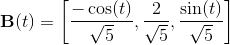# Calculus 3 : Binormal Vectors

## Example Questions

### Example Question #1 : Binormal Vectors

Find the binormal vector of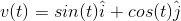.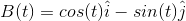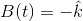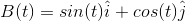Does not exist.Explanation:

To find the binormal vector, you must first find the unit tangent vector, then the unit normal vector.

The equation for the unit tangent vector,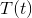,  is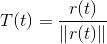where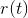is the vector and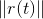is the magnitude of the vector.

The equation for the unit normal vector,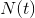,  is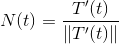where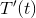is the derivative of the unit tangent vector and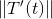is the magnitude of the derivative of the unit vector.

The binormal vector is the cross product of unit tangent and unit normal vectors, or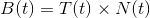For this problem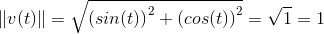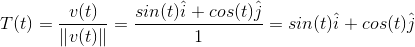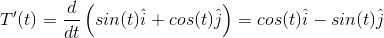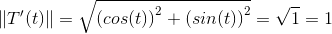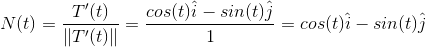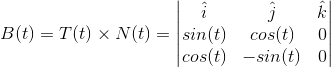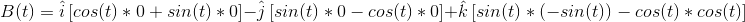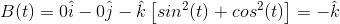### Example Question #2 : Binormal Vectors

Find the binormal vector of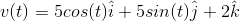.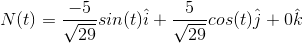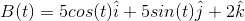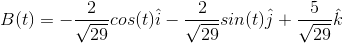Does not existExplanation:

To find the binormal vector, you must first find the unit tangent vector, then the unit normal vector.

The equation for the unit tangent vector,,  iswhereis the vector andis the magnitude of the vector.

The equation for the unit normal vector,,  iswhereis the derivative of the unit tangent vector andis the magnitude of the derivative of the unit vector.

For this problem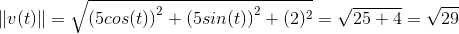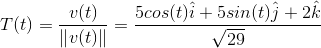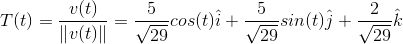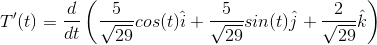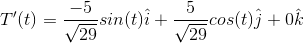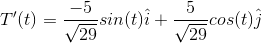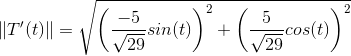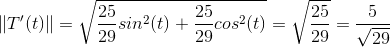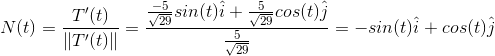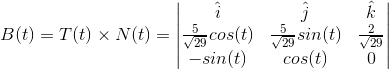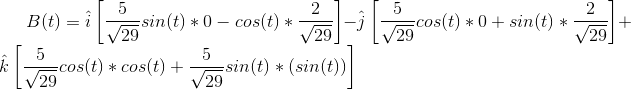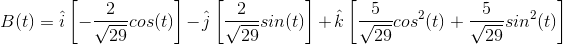### Example Question #3 : Binormal Vectors

Find the binormal vector for: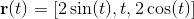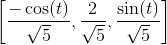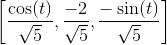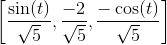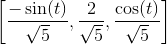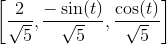Explanation:

The binormal vector is defined as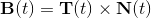Where T(t) (the tangent vector) and N(t) (the normal vector) are: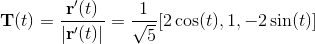and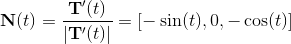The binormal vector is: